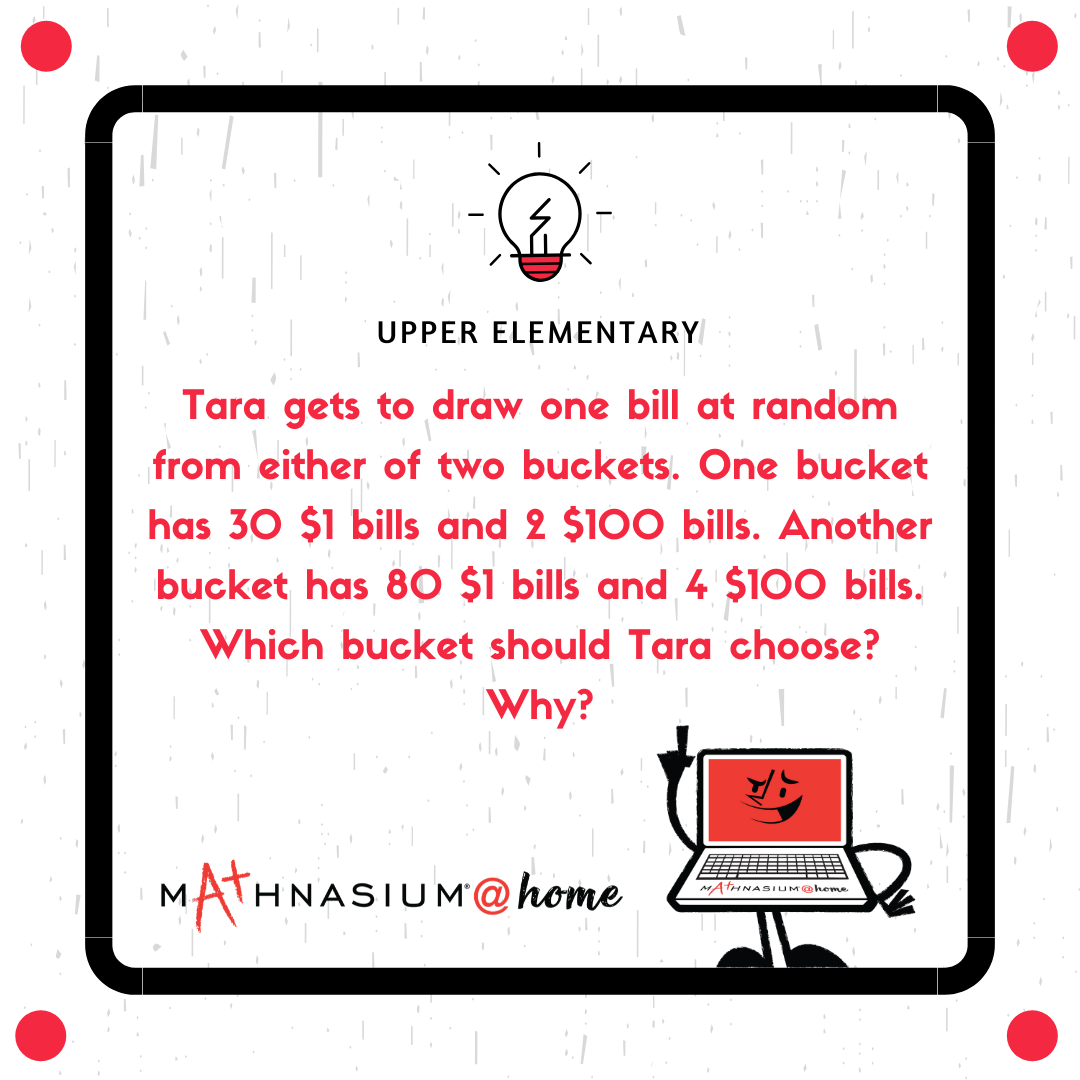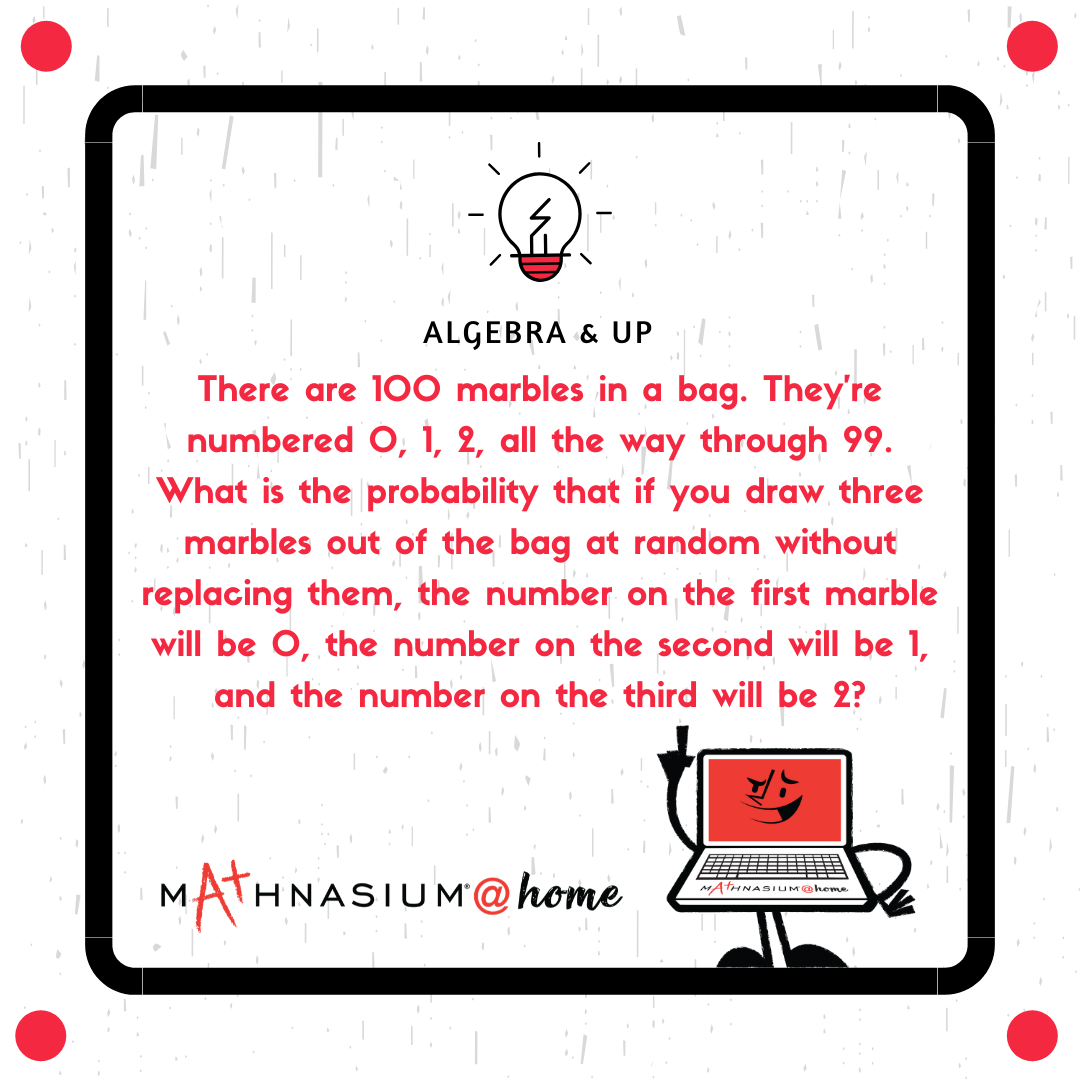877-601-6284
Get Started by Finding a Local Center

# Word Problems: April 5th - 11th

Apr 5, 2020 | Cave Creek

# At home with students looking for an educational distraction? Look no further! Find your child's grade level and work through the problems with them!## Solution:

It’s as likely as not that Martin rolls an even number because there are 3 even numbers and 3 odd numbers on a die. It’s impossible that he rolls an 8 because there isn’t an 8 on a die. It’s certain that he rolls a number less than 10 because all the numbers on a die are less than 10. It’s unlikely that he rolls a 1 because only 1 out of 6 numbers on a die is 1. It’s very likely that he doesn’t roll a 6 because 5 out of 6 numbers on a die aren’t 6.## Solution:

Since 2 out of 30 + 2 = 32 bills in the first bucket are \$100 bills, that means that 2/32 = 1/16 of the bills are \$100. In the other bucket, 4 out of 80 + 4 = 84 bills are \$100, so 4/84 = 1/21 bills are \$100. Since the first bucket has the greater fractional part comprising \$100 bills, that’s the smarter bucket to choose.## Solution:

There are 7 + 18 = 25 fruits in the box. Since 18 out of 25 fruits are oranges and a percentage is out of 100, we can multiply 18 and 25 each by 4 to find the number of fruits out of 100 that are oranges. 18 × 4 = 72, so 72% of the fruits are oranges. If one is chosen at random, then the percent chance that it’ll be an orange is 72%.## Solution:

The probability of drawing the 0 first is 1/100. If the marble isn’t replaced, then the probability of drawing the 1 next is 1/99. Then, the probability of drawing the 2 next is 1/98. So, the probability of drawing exactly those numbers in that order is 1/(100 × 99 × 98) = 1/970,200.

## Get the same great Mathnasium Experience from the comfort of your home.

• You get the same instructors and Mathnasium Method(TM) you know from our center, face-to-face, online and in real-time.

• Learning can happen anywhere there's an internet connection.

• It's safe and secure!

## (602) 313-0901 [email protected]##### Locations near
Could not find Center, try again